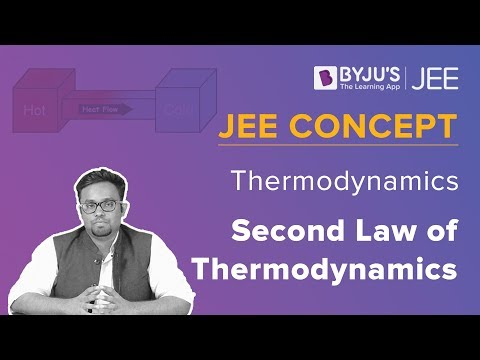# CBSE Class 11 Physics Notes Thermodynamics

According to the CBSE Syllabus 2023-24, this chapter has been renumbered as Chapter 11.

## What is Thermodynamics?

Thermodynamics is concerned with the relationship between other forms of energy and heat. It explains how thermal energy is converted from other forms of energy. The energy coming from heat is termed Thermal energy. When the tiny particles within an object move, they generate heat, and more amount of heat is generated when these particles move at a faster pace.### What are the Laws of Thermodynamics?

The laws of thermodynamics define the fundamental physical quantities like energy, temperature, and entropy that characterize thermodynamic systems at thermal equilibrium. The four laws of thermodynamics are:

• Zeroth Law: It states that when two systems are in thermal equilibrium with the third system which is separate, the whole system together is said to be in equilibrium with one another.
• First Law: It states the law of conservation of energy when applied to a system in which the energy transfer occurs from or towards the system (with the help of heat and work). It represents the relation between the work done by the system, the heat supplied to the system and the change in the internal energy of the system.
• Second Law: It states that it is not possible to find a system, where the absorption of heat from the reservoir is the complete conversion of heat into work.
• Third Law: This law states that at an absolute zero temperature, the system possesses minimum energy.### What is Enthalpy?

In a thermodynamic setup, enthalpy is the measurement of energy. The quantity of enthalpy equals the total content of the heat of a system, equivalent to the system’s internal energy plus the product of volume and pressure.

Numerically, H (the enthalpy), equals the sum of E (the internal energy), P (the product of the pressure), and V (volume) of the system.

H = E + PV

### What is Entropy?

Entropy’s value depends on the physical state or condition of a system. It is a thermodynamic function that is utilized to measure the disorder or randomness of a system. For instance, the entropy of a solid, where particle movement is restricted, is less than the entropy of a gas, wherein the particles occupy the container.

 Also Access NCERT Solutions for Class 11 Physics Chapter 12 NCERT Exemplar for Class 11 Physics Chapter 12

### Important Questions

1. Calculate the coefficient of performance when a refrigerator maintains a temperature of 9 degrees Celsius and the room temperature is said to be 36 degrees.
2. An electric heater provides heat to a system which is stated to be 1000W. Calculate the increase in the rate of internal energy when the system performs work at a rate of 65 joules per second.
3. The amount of work done on a system in order to change the state of gas adiabatically from equilibrium A to B is 33.2 J. If the gas is taken from A to B from a method in which the net heat absorbed is 9.35 cal. Calculate the work done by the system. (assume 1 cal=4.19 J)

Learn in detail about class 11 chapter 12 Thermodynamics and more at BYJU’S.

 Zeroth Law of Thermodynamics Second Law Of Thermodynamics First Law Of Thermodynamics Third Law Of Thermodynamics

## Frequently Asked Questions on CBSE Class 11 Physics Notes Chapter 12 Thermodynamics

Q1

### How many laws of thermodynamics are there?

Thermodynamics has recognised three fundamental laws, named by an ordinal identification, the first law, the second law, and the third law.

Q2

### What is entropy?

Entropy is the measure of a system’s thermal energy per unit temperature that is unavailable for doing useful work.

Q3

### What is thermal energy?

Thermal energy is a type of power produced by atomic and molecular particle movement within a substance.